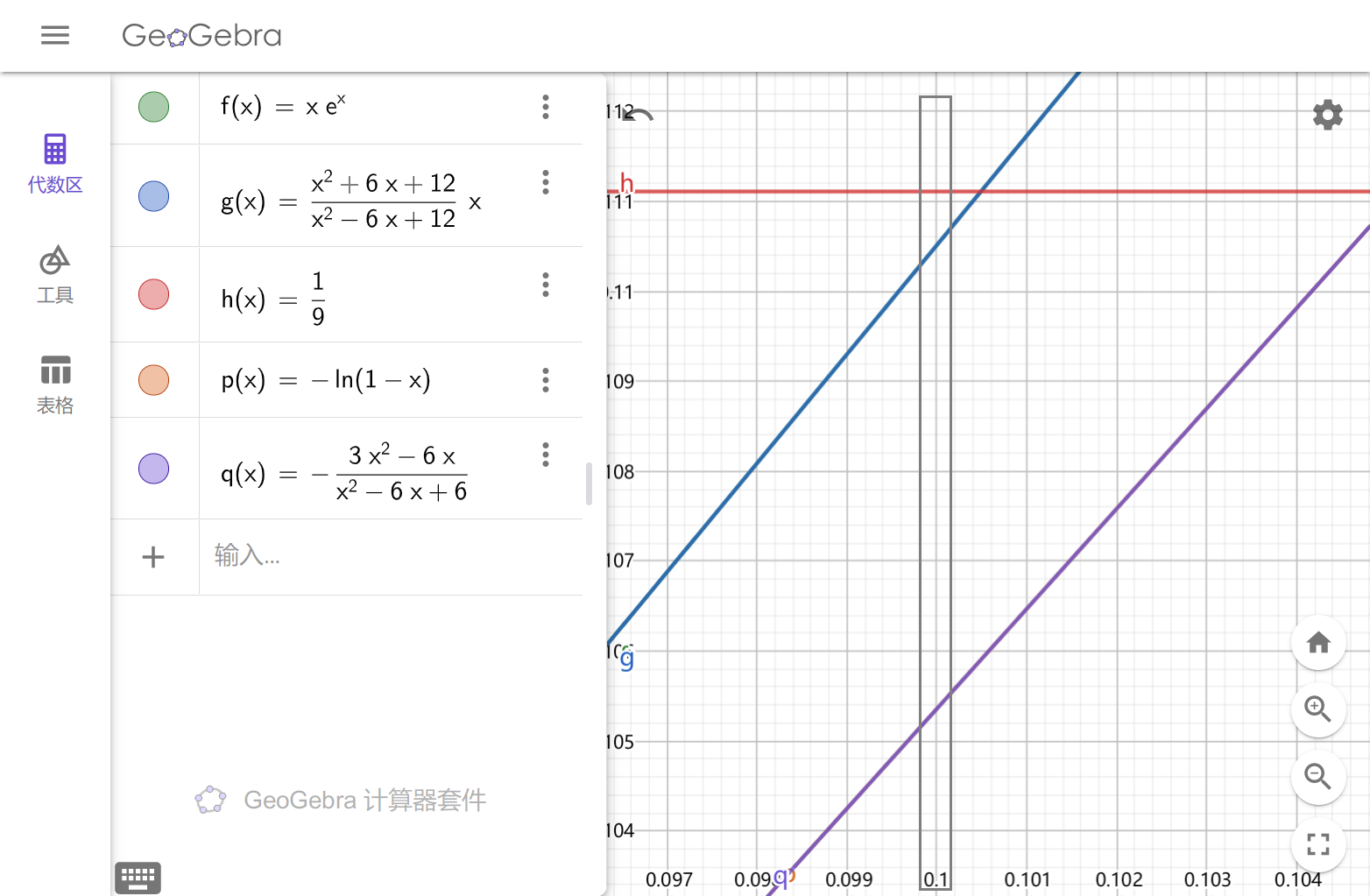07/16/2022

2022 年新高考 I 卷中出现的比较 $a=0.1e^{0.1}$$b=\dfrac 19$$c=-\ln 0.9$ 三个数大小的选择题即为这种方法的典型例题。

## 切线放缩

\begin{aligned} e^x\ge x+1 &\Leftrightarrow \ln(x+1) \le x \\ \ln x\le x-1 &\Leftrightarrow e^x\ge ex \end{aligned}

## 泰勒展开

#### 应用：得到常用不等式

$e^x=1+x+\dfrac{x^2}{2!}+\dfrac{x^3}{3!}+\dfrac{x^4}{4!}+\cdots$ 我们可以得到 $e^x\ge x+1,\quad e^x\ge \dfrac{x^2}{2} +x+1$ 等不等式。

## 帕德逼近

$e^x$ $m=0$ $m=1$ $m=2$
$n=0$ $1$ $\dfrac{x+1}{1}$（恒小于） $\dfrac{x^2+2x+2}{2}$
$n=1$ $\dfrac{1}{-x+1}$（恒大于） $\dfrac{x+2}{-x+2}$ $\dfrac{x^2+4x+6}{-2x+6}$（恒大于）
$n=2$ $\dfrac{2}{x^2-2x+2}$ $\dfrac{2x+6}{x^2-4x+6}$（恒小于） $\dfrac{x^2+6x+12}{x^2-6x+12}$
$\ln(x+1)$ $m=1$ $m=2$
$n=0$ $x$（恒小于） $\dfrac{2x-x^2}{2}$
$n=1$ $\dfrac{2x}{x+2}$ $\dfrac{x^2+6x}{4x+6}$（恒大于）
$n=2$ $\dfrac{2x}{x+2}$（恒大于） $\dfrac{3x^2+6x}{x^2+6x+6}$

#### 应用：一类“比大小”问题

2022 年新高考全国 I 卷 7.$a=0.1e^{0.1}$$b=\dfrac 19$$c=-\ln 0.9$，则（$\quad$

A. $a B. $c C. $c D.$a

\left\{\begin{aligned} 10\cdot a &= \frac{0.01+0.6+12}{0.01-0.6+12} = \frac{12.61}{11.41} \\ 10\cdot b &= \frac{10}{9} \\ 10\cdot c &= \frac{0.6-0.03}{0.01-0.6+6} = \frac{5.7}{5.41} \\ \end{aligned}\right.#### 应用：手算 $\ln(x)$ 的值

$x$ 拆分成 $x=2\cdot \dfrac{c_1+1}{c_1}\cdot \dfrac{c_2+1}{c_2}\cdot \dfrac{c_3+1}{c_3} \cdots$ 的形式，然后代入 $\ln 2=0.693$$\ln\left(\dfrac{n+1}{n}\right)\sim\dfrac{2}{2n+1}\quad (n\ge 3)$ 得近似值。

\begin{aligned} \ln 11 &= \ln \dfrac {11}{10} + \ln 5 + \ln 2 \\ &= \ln \dfrac {11}{10} + \ln {5}{4} + 3\ln 2 \\ &\approx \frac {2}{21} +\frac {2}{9}+3\times 0.693 \approx 2.3964) \end{aligned}